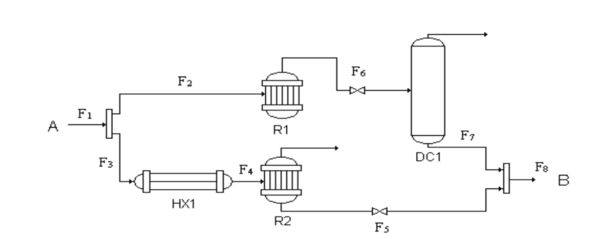# Convex generalized disjunctive programming (GDP)

Edited By: Nicholas Schafhauser, Blerand Qeriqi, Ryan Cuppernull

## Introduction

Generalized disjunctive programming (GDP) involves logic propositions (Boolean variables) and sets of constraints that are chained together using the logical OR. In order to take advantage of current mixed-integer nonlinear programming solvers (e.g. DICOPT (Viswanathan and Grossmann, 1990), SBB (Brooke et al., 1998), α-ECP (Westerlund and Pettersson, 1995), Bonmin (Bonami et al., 2008), FilMINT (Abhishek et al., 2006), BARON (Sahinidis, 1996), etc.), GDPs are often reformulated as MINLPs.

## Theory

The general form of an MINLP model is as follows

{\begin{aligned}\min z=f(x,y)\\s.t.g(x,y)\leq 0\\x\in X\\y\in Y\\\end{aligned}}where f(x) and g(x) are twice differentiable functions, x are the continuous variables and y are the discrete variables. There are three main types of sub problems that arise from the MINLP: Continuous Relaxation, NLP subproblem for a fix yP and the feasibility problem.

#### Continuous Relaxation

The sub problem of continuous relaxation takes the form of

{\begin{aligned}\min z=f(x,y)\\s.t.g(x,y)\leq 0\\x\in X\\y\in Y_{R}\\\end{aligned}}Where $Y_{R}$is the continuous relaxation of Y. Not that in this sub-problem all of the integer variables y are treated as continuous. This also returns a Lower Bound when it returns a feasible solution

#### NLP Subproblem for a fixed yP

The subproblem for a fixed yP is shown in the form below

{\begin{aligned}\min z=f(x,y^{p})\\s.t.g(x,y^{p})\leq 0\\x\in \Re ^{n}\\\end{aligned}}In this sub problem you return an upper bound for the MINLP program when it has a feasible solution. So with said you can fix on of these integer variables and continuously relax the others in order to get a range of feasible values.

Feasibility Problem

When the fixed MINLP subproblem is not feasible the following feasibility problem is considered.

{\begin{aligned}\min z=f(x,y)\\s.t.g(x,y)\leq 0\\j\in J\\u\in \Re \\\end{aligned}}Where J is the index set for inequalities and the feasibility problem attempts to minimize the infeasibility of the solution with the most violated constraints.

#### GDP

Generalized Disjunctive Programming provides a high level framework for solving the mixed non-linear integer programs. By provide a methodology for converting the dijunctive problems into a MINLP the problem becomes simplified and easier to solve using current processing and algorithmic capabilities. There a methodologies that can not only solve this both Convex and Non-Convex Problems. A Convex GDP is when both f(x) and g(x) are convex functions. Which is defined as a graph where any line segment that passes through any 2 points of the plot will always be greater than the plot itself. This allows for simple relaxations/approximations to occur which will create a faster solving methodology.

## Methodology

The two most common ways of reformulating a GDP problem into an MINLP are through Big-M (BM) and Hull Reformulation (HR). BM is the simpler of the two, while HR results in tighter relaxation (smaller feasible region) and faster solution times.

Below is an example of the reformulation of the GDP problem from the Theory section reformulated into an MINLP by using the Big-M method.

{\begin{aligned}\min z=f(x)\\s.t.g(x)\leq 0\\r_{ki}(x)\leq M^{ki}(1-y_{ki})\quad k\in K,i\in D_{k}\\\sum _{i\in D_{k}}y_{ki}=1\quad k\in K\\Hy\geq h\\x^{lo}\leq x\leq x^{up}\\x\in \Re ^{n}\\y_{ki}\in {0,1}\quad k\in K,i\in D_{k}\end{aligned}}Notice that the boolean term from the original GDP has been converted into a numerical {0,1}. The logic relations have also been converted into linear integer constraints (Hy).

This MINLP reformulation can now be used in well-known solvers to calculate a solution.

#### Solvers:

• DICOPT
• AAOA
• BARON
• Couenne
• Partial list taken from: http://egon.cheme.cmu.edu/Papers/IMAGrossmannRuiz.pdf

## Numerical Example

The following example was taken from: http://egon.cheme.cmu.edu/Papers/IMAGrossmannRuiz.pdf

## Applications

GDP formulations are useful for real-world applications where multiple branches are available when making decisions. Solving the GDP in these instances will allow the user to calculate which decisions should be made at each branching point in order to get the optimal solution. This disjunctive formulation is common in complex chemical reactions and production planning.Figure 2: Process Network Example. Each decision point represents another disjunctive set. 

The process network depicted in the Figure 2 depicts multiple decisions that could be made to all end up at the goal (B) in a chemical reaction. This problem is able to be formulated into a GDP in order to figure out which route should be taken in order to maximize the profit.

This same idea can be scaled to larger problems with more complex branching. Figure 3 illustrates a larger process network and all of the different decision points. This problem is able to be formulated into a GDP so that the most optimal route can be calculated to take through the network.

## Conclusion

GDP is a programming method that applies disjunctive programming to MINLP problems. This method facilitates modeling discrete or continuous optimization problems by implementing algebraic constraints and logic expressions. The formulation of a GDP consists of Boolean and continuous variables and disjunctions and logic propositions. In the case of convex functions, GDPs can be reformulated using the big-M and the hull relaxation. Formulation methods also include logic based methods disjunctive branch and bound and decomposition. Once reformulated into a standard MINLP, standard MILNP solvers, such as DICOPT, SBB, α-ECP and BARON, can be used to determine optimal solutions. The GDP method has important applications that include the optimization of complex chemical reactions and process planning.